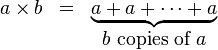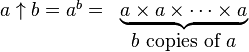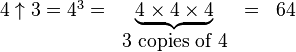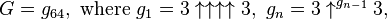Graham’s Number – literally big enough to collapse your head into a black hole

Graham’s Number is a number so big that it would literally collapse your head into a black hole were you fully able to comprehend it. And that’s not hyperbole – the informational content of Graham’s Number is so astronomically large that it exceeds the maximum amount of entropy that could be stored in a brain sized piece of space – i.e. a black hole would form prior to fully processing all the data content. This is a great introduction to notation for really big numbers. Numberphile have produced a fantastic video on the topic:

Graham’s Number makes use of Kuth’s up arrow notation (explanation from wikipedia:)

In the series of hyper-operations we have

1) Multiplication:For example,2) Exponentiation:For example,3) Tetration:For example,etc.

4) Pentation:and so on.

Examples:Which clearly can lead to some absolutely huge numbers very quickly. Graham’s Number – which was arrived at mathematically as an upper bound for a problem relating to vertices on hypercubes is (explanation from Wikipedia)where the number of arrows in each layer, starting at the top layer, is specified by the value of the next layer below it; that is,and where a superscript on an up-arrow indicates how many arrows are there. In other words, G is calculated in 64 steps: the first step is to calculate g1 with four up-arrows between 3s; the second step is to calculate g2 with g1 up-arrows between 3s; the third step is to calculate g3 with g2 up-arrows between 3s; and so on, until finally calculating G = g64 with g63 up-arrows between 3s.

So a number so big it can’t be fully processed by the human brain.  This raises some interesting questions about maths and knowledge – Graham’s Number is an example of a number that exists but is beyond full human comprehension – it therefore is an example of a upper bound of human knowledge.  Therefore will there always be things in the Universe which are beyond full human understanding?  Or can mathematics provide a shortcut to knowledge that would otherwise be inaccessible?

If you enjoyed this post you might also like:

How Are Prime Numbers Distributed? Twin Primes Conjecture – a discussion about the amazing world of prime numbers.

Wau: The Most Amazing Number in the World? – a post which looks at the amazing properties of Wau